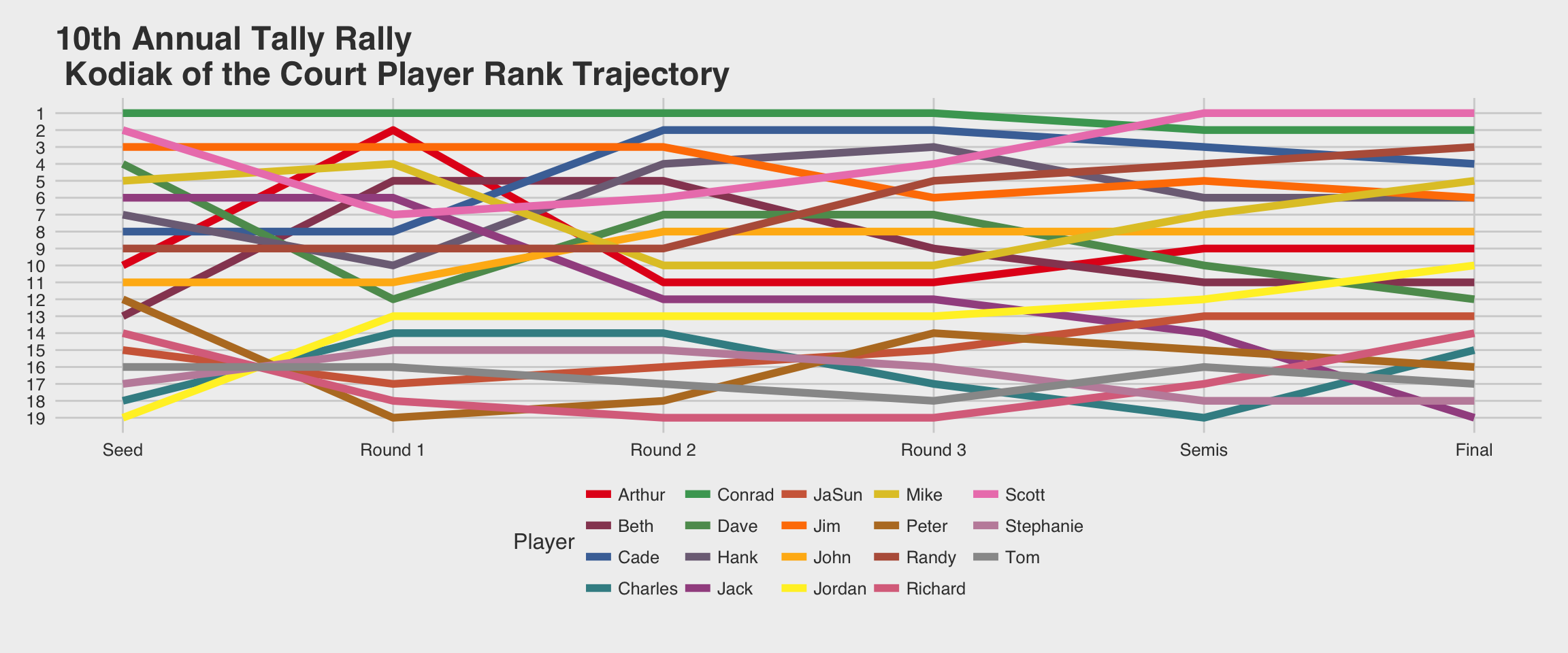# Spring Escape

Last month I organized the 10th annual Tally Rally frisbee tournament featuring top players from around the country including as far away as Wisconsin, Alaska, California, and Virginia. The game is officially called Double Disc Court, but informally we call it `Escape.’ The game is played like doubles tennis except that there are two discs and you and your teammate need to avoid getting caught with both discs at the same time. See https://youtu.be/nszaRylOxus for more details.

But the point here is to demonstrate how to create line graphs in R without legends. Starting with an initial seeding of players the tournament proceeds in rounds with players moving up or down in ranking according to how well they perform. A graph of the rank by round provides a rank trajectory through the tournament by player. Perhaps you can use graphs like these?

``````nP = 19
Round = 0:5
Conrad = c(1, 1, 1, 1, 2, 2)
Scott = c(2, 7, 6, 4, 1, 1)
Jim = c(3, 3, 3, 6, 5, 6)
Dave = c(4, 12, 7, 7, 10, 12)
Mike = c(5, 4, 10, 10, 7, 5)
Jack = c(6, 6, 12, 12, 14, 19)
Hank = c(7, 10, 4, 3, 6, 6)
Cade = c(8, 8, 2, 2, 3, 4)
Randy = c(9, 9, 9, 5, 4, 3)
Arthur = c(10, 2, 11, 11, 9, 9)
John = c(11, 11, 8, 8, 8, 8)
Peter = c(12, 19, 18, 14, 15, 16)
Beth = c(13, 5, 5, 9, 11, 11)
Richard = c(14, 18, 19, 19, 17, 14)
JaSun = c(15, 17, 16, 15, 13, 13)
Tom = c(16, 16, 17, 18, 16, 17)
Stephanie = c(17, 15, 15, 16, 18, 18)
Charles = c(18, 14, 14, 17, 19, 15)
Jordan = c(19, 13, 13, 13, 12, 10)

Wide = data.frame(Round, Conrad, Scott, Jim, Dave, Mike, Jack, Hank, Cade, Randy,
Arthur, John, Peter, Beth, Richard, JaSun, Tom, Stephanie, Charles, Jordan)``````
``````colnames(Wide) = c(Round, "Conrad Damon", "Scott Zimmerman", "Jim Elsner", "Dave Taylor", "Mike Prost",
"Jack Cooksey", "Hank Bass", "Cade Loving", "Randy Aiu", "Arthur Coddington",
"John Elsner", "Peter Andrews", "Beth Verish", "Richard Paschal", "JaSun Burdick",
"Tom McMullen", "Stephanie Chan", "Charles Robshaw", "Jordan Huston")``````

Wide to long

``````library("tidyr")
library("ggplot2")
library("ggthemes")
Long = gather(Wide, Player, Rank, 2:20)

library("RColorBrewer")
getPalette = colorRampPalette(brewer.pal(9, "Set1"))

ggplot(Long, aes(x = Round, y = Rank, color = Player)) +
geom_line(size = 2) +
xlab("") +
scale_x_continuous(breaks = 0:5, labels = c("Seed", "Round 1", "Round 2", "Round 3", "Semis", "Final")) +
scale_y_reverse(breaks = 1:19) +
ylab("Rank") +
theme_fivethirtyeight() +
ggtitle("10th Annual Tally Rally\n Kodiak of the Court Player Rank Trajectory") +
scale_color_manual(values = getPalette(nP))````````````ggplot(Long, aes(x = Round, y = Rank, color = Player)) +
geom_line(size = 2) +
xlab("") + ylab("") +
scale_x_continuous(breaks = 0:5, labels = c("Seed", "Round 1", "Round 2", "Round 3", "Semis", "Final")) +
scale_y_reverse(breaks = 1:19, labels = (unique(Long\$Player))) +
theme_economist() +
ggtitle("10th Annual Tally Rally\n Player Rank Trajectory") +
scale_color_manual(values = getPalette(nP), guide = FALSE)``````